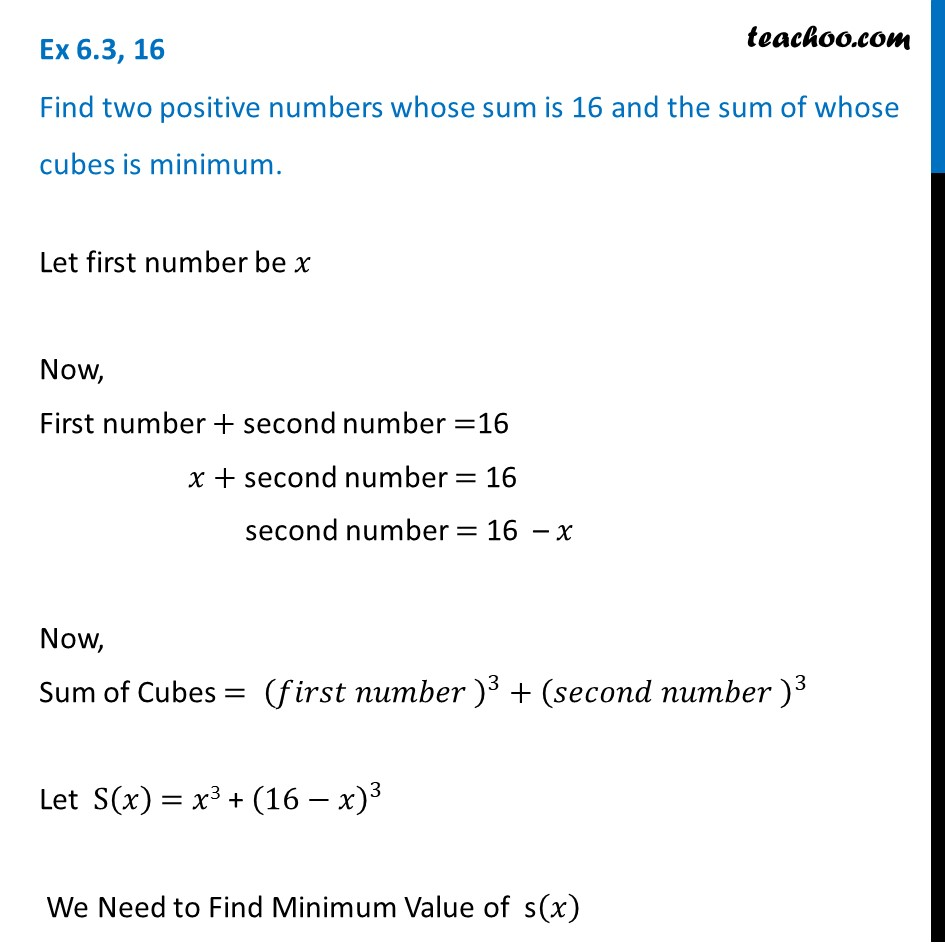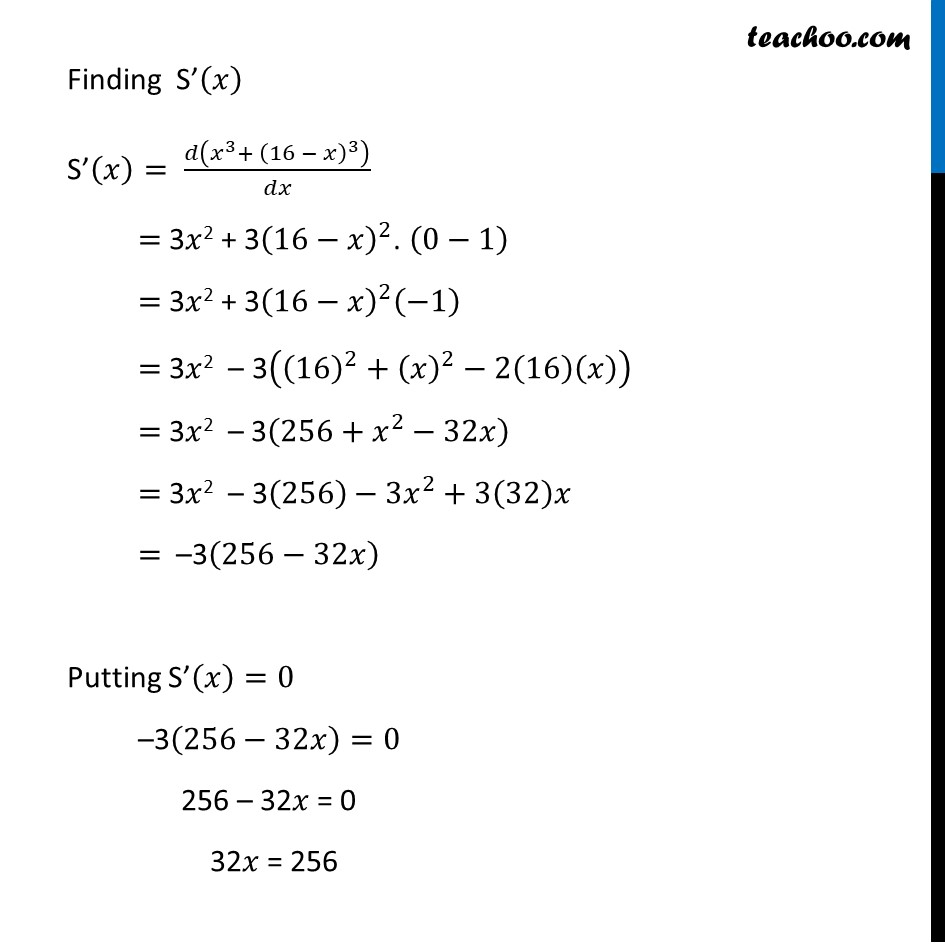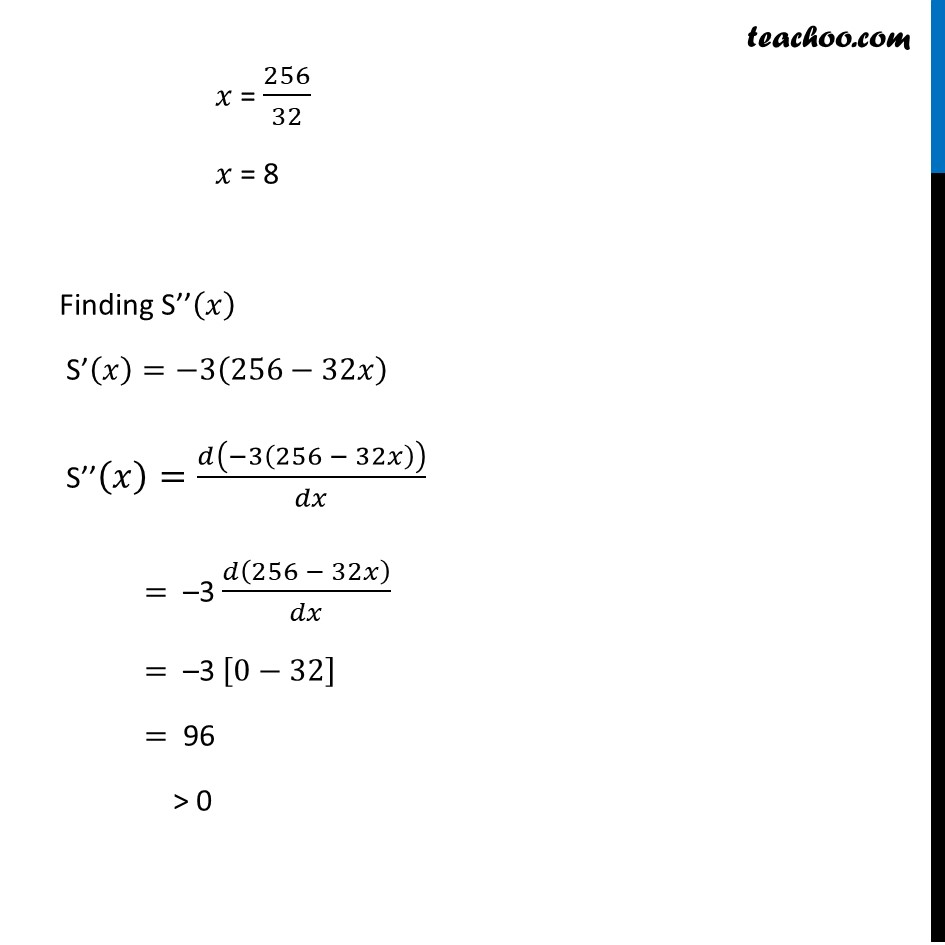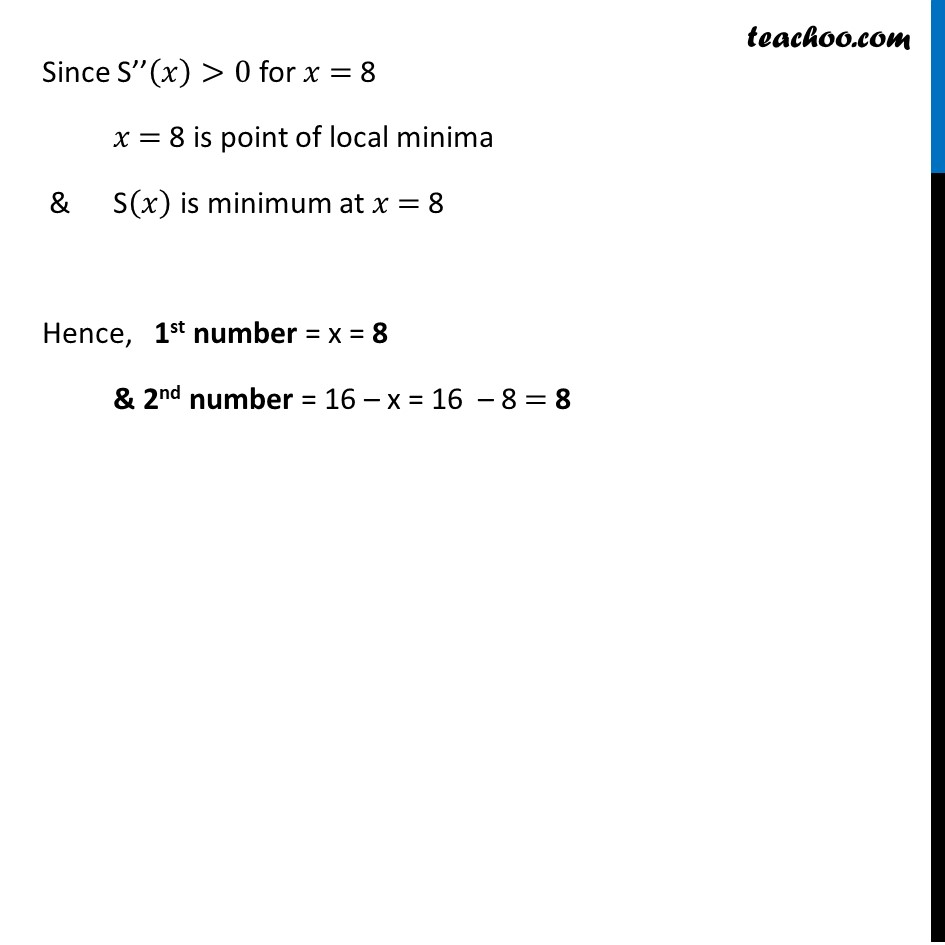Ex 6.3

Chapter 6 Class 12 Application of Derivatives
Serial order wiseLearn in your speed, with individual attention - Teachoo Maths 1-on-1 Class

### Transcript

Ex 6.3, 16 Find two positive numbers whose sum is 16 and the sum of whose cubes is minimum.Let first number be 𝑥 Now, First number + second number =16 𝑥 + second number = 16 second number = 16 – 𝑥 Now, Sum of Cubes = (𝑓𝑖𝑟𝑠𝑡 𝑛𝑢𝑚𝑏𝑒𝑟 )^3+(𝑠𝑒𝑐𝑜𝑛𝑑 𝑛𝑢𝑚𝑏𝑒𝑟 )^3 Let S(𝑥) = 𝑥3 + (16−𝑥)^3 We Need to Find Minimum Value of s(𝑥) Finding S’(𝑥) S’(𝑥)= 𝑑(𝑥^3+ (16 − 𝑥)^3 )/𝑑𝑥 = 3𝑥2 + 3(16−𝑥)^2. (0−1) = 3𝑥2 + 3(16−𝑥)^2 (−1) = 3𝑥2 – 3((16)^2+(𝑥)^2−2(16)(𝑥)) = 3𝑥2 – 3(256+𝑥^2−32𝑥) = 3𝑥2 – 3(256)−3𝑥^2+3(32)𝑥 = –3(256−32𝑥) Putting S’(𝑥)=0 –3(256−32𝑥)=0 256 – 32𝑥 = 0 32𝑥 = 256 𝑥 = 256/32 𝑥 = 8 Finding S’’(𝑥) S’(𝑥)=−3(256−32𝑥) S’’(𝑥)=𝑑(−3(256 − 32𝑥))/𝑑𝑥 = –3 𝑑(256 − 32𝑥)/𝑑𝑥 = –3 [0−32] = 96 > 0 Since S’’(𝑥)>0 for 𝑥 = 8 𝑥 = 8 is point of local minima & S(𝑥) is minimum at 𝑥 = 8 Hence, 1st number = x = 8 & 2nd number = 16 – x = 16 – 8 = 8